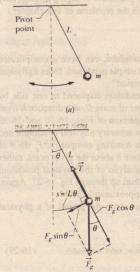# The Simple Pendulum

The Simple Pendulum

If you hang an apple at the end of a long thread fixed at its upper end. and then set the apple swinging back and forth a small distance, you easily see that the apple’s motion is periodic. Is it. in fact. simple harmonic motion If so what is the period T  To answer we consider a simple pendulum, which consists of a particle of mass III (called the bob of the pendulum) suspended from one end of an unstretchable. massless string of length L that is fixed at the other end. as in . The bob is free to swing back and forth in the plane of the page to the left and right of a vertical line through the pendulum’s pivot point.  The forces acting on the bob are the force T from the string and the gravitational force  as show where the string makes an angle e with the vertical. We resolve F. into a radial component Fg cos e and a component  sin e that is tangent to the path taken by the bob. This tangential component produces a restoring torque about the pendulum’s pivot point. because it always acts opposite the displacement of the bob so as to bring the bob back toward its central location.

That location is called the equilibrium position (e = 0). because the pendulum would be at rest there were it not swinging (T = r l.F). we can write this restoring torque as T = -L(F sin e).  where the minus sign indicates that the torque acts to reduce e. and L is the moment arm of the force component  e about the pivot point. Substituting  into (T = la) and then substituting mg as the magnitude of  we obtain -L(mg sin e) = l a,   where I is the pendulum’s rotational inertia about the pivot point and a is its angular acceleration about that point. We can simplify  if we assume the angle e is small. for then we can approximate sin e with e (expressed in radian measure). (As an example. if e = 5.000 =  .0873 rad, then sin e = 0.0872, a difference of only about O.I%.) With that approximation and some rearranging. we then have  mgL a= —8J .

This equation is the angular equivalent of the hallmark of SHM. It tellsus tnat the angular acceleration a of the pendulum is proportional to the angul displacement 8 but opposite in sign. Thus, as the pendulum bob moves to, say, tI right as in  its acceleration to the left increases until it stops and begil moving to the left. Then, when it is on the left, its acceleration to the right tends return it to the right, and so on, as it swings back and forth in SHM. More precise the motion of a simple pendulum swinging through only small angles is approx mately SHM. We can state this restriction to small angles another way: The angul amplitude 8″, of the motion (the maximum angle of swing) must be smaH. Comparing and we see that the angular frequency the pendulum is w = J mgUI. Next, if we substitute this expression for w in  (w = 21TIT), we see that the period of the pendulum may be written as T =. 21TJ mg1L .A simple pendulum. (b) The forces acting on the bob are the gravitational force iZ and the force f from the string. The tangential cornponent F, sin (}of the gravitational force is a restoring force that tends to bring the pendulum back to its central position.

An the mass of a simple pendulum is concentrated in the mass m of the particl like bob, which is at radius L from the pivot points we can use (I = mr2) to write 1 = mL2 for the rotational inertia of the pendulum. Subsdtu ing this into and simplifying then yield

T = 21TJ”f. as a simpler expression for the period of a simple pendulum swinging through on small angles. (We assume small-angle swinging in the problems of this.